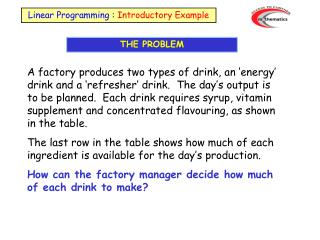DownloadDownload PresentationTHE PROBLEM

# THE PROBLEM

Télécharger la présentation## THE PROBLEM

- - - - - - - - - - - - - - - - - - - - - - - - - - - E N D - - - - - - - - - - - - - - - - - - - - - - - - - - -
##### Presentation Transcript

1. Linear Programming : Introductory Example THE PROBLEM A factory produces two types of drink, an ‘energy’ drink and a ‘refresher’ drink. The day’s output is to be planned. Each drink requires syrup, vitamin supplement and concentrated flavouring, as shown in the table. The last row in the table shows how much of each ingredient is available for the day’s production. How can the factory manager decide how much of each drink to make?

2. THE PROBLEM Energy drink sells at £1 per litre Refresher drink sells at 80 p per litre

3. FORMULATION Syrup constraint: Let x represent number of litres of energy drink Let y represent number of litres of refresher drink 0.25x + 0.25y 250  x + y 1000

4. FORMULATION Vitamin supplement constraint: Let x represent number of litres of energy drink Let y represent number of litres of refresher drink 0.4x + 0.2y 300 2x + y 1500

5. FORMULATION Concentrated flavouring constraint: Let x represent number of litres of energy drink Let y represent number of litres of refresher drink 6x + 4y 4800 3x + 2y 2400

6. FORMULATION • Objective function: • Let x represent number of litres of energy drink • Energy drink sells for £1 per litre • Let y represent number of litres of refresher drink • Refresher drink sells for 80 pence per litre • Maximise x + 0.8y

7. SOLUTION Empty grid to accommodate the 3 inequalities

8. SOLUTION 1st constraint Draw boundary line: x + y = 1000

9. SOLUTION 1st constraint Shade out unwanted region: x + y  1000

10. SOLUTION Empty grid to accommodate the 3 inequalities

11. SOLUTION 2nd constraint Draw boundary line: 2x + y = 1500

12. SOLUTION 2nd constraint Shade out unwanted region: 2x+ y 1500

13. SOLUTION Empty grid to accommodate the 3 inequalities

14. SOLUTION 3rd constraint Draw boundary line: 3x + 2y = 2400

15. SOLUTION 3rd constraint Shade out unwanted region: 3x + 2y 2400

16. SOLUTION All three constraints: First: x + y 1000

17. SOLUTION All three constraints: First: x + y 1000 Second: 2x + y  1500

18. SOLUTION All three constraints: First: x + y 1000 Second: 2x + y 1500 Third: 3x + 2y 2400

19. SOLUTION All three constraints: First: x + y 1000 Second: 2x + y 1500 Third: 3x + 2y 2400 Adding: x 0 and y 0

20. SOLUTION Feasible region is the unshaded area and satisfies: x + y 1000 2x + y 1500 3x + 2y 2400 x  0 and y 0

21. SOLUTION Evaluate the objective function x + 0.8y at vertices of the feasible region: O: 0 + 0 = 0 A: 0 + 0.8x1000 = 800 B: 400 + 0.8x600 = 880 C: 600 + 0.8x300 = 840 D: 750 + 0 = 750 A B C D O Maximum income = £880 at (400, 600)

22. SOLUTION Alternatively, draw a straight line x + 0.8y = k. A Move a ruler parallel to this line until it reaches the edge of the feasible region. B The furthest point you can move it to is point B. C D At B (400, 600) the value of the objective function is 880. O Maximum income = £880 at (400, 600)